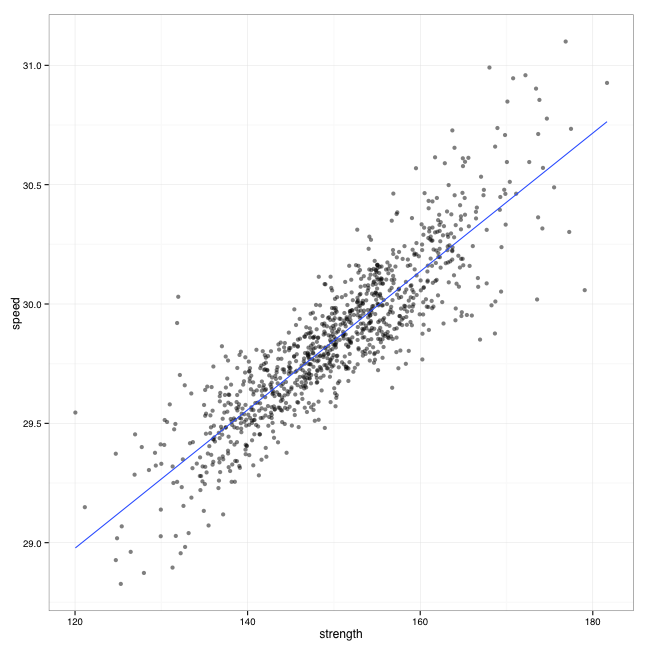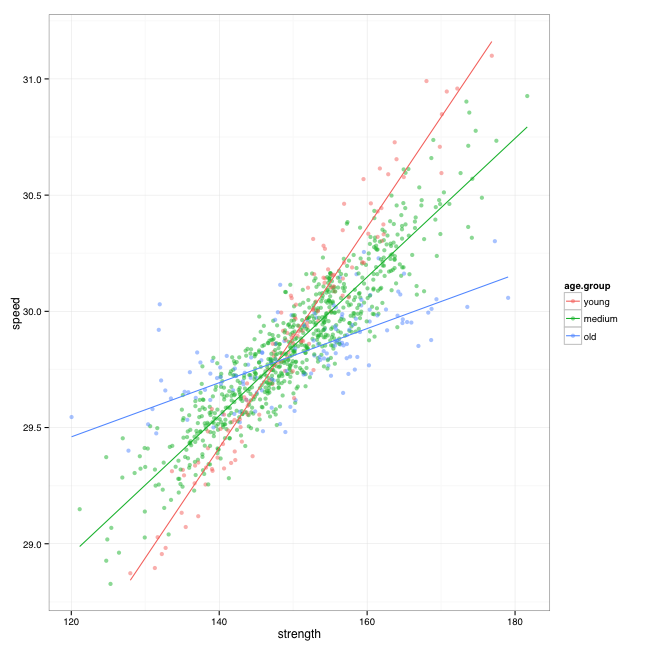## Tuesday, December 31, 2013

### Playbook: Understanding MODERATION through simulation

Playbook: Understanding MODERATION through simulation

# Playbook: Understanding MODERATION through simulation

## Introduction

I recently spoke with my college professor regarding the understanding of statistics, and I remarked that I learn the statistical concept the best (and with full comprehension) through simulation. He remarked that I might be in the minority of students (p<0.01 - see what I did here?). Not sure if this is true - if one day I manage to teach introductory course on statistics I will for sure try to use simulation and guided discovery.

Most of the statistical concepts seems to really dig in in my case when I simulate the data and try to find out how the statistical tests (and linear models) try to re-create the simulated relationships. Will Hopkins make a wonderful tutorial on using simulation HERE which I refer to now and then.

Anyway, I was going through the great FREE course Statistics One by prof. Andrew Conway at Coursera and I am trying to understand the hardest lectures on Moderation and Mediation. Because the concepts couldn't dig in, I decided to play with simulation. No, not stimulation - SIMULATION (Note to myself: too much reading of Andy Field Discovering Statistics Using R and buying in to his type of humor)

In the following post I will try to simulate the data for moderation analysis. So this is my playbook using simulation and if anyone finds it useful great, if not, just disregard it. To find more about moderation please take a look at Wiki page) and mentioned Coursera course.

## Simulating the data

To simulate the data I decided to use the relationship between squat strength and maximal velocity. Let's suppose that the more one squats the higher speed one can reach. In this case predictor variable is squat strength and outcome variable is maximal speed.

Now we introduce the moderator variable: AGE. Let's assume that the older the athlete the less transfer there is and vice versa (i.e. increase in strength will yield less increase in speed with older athletes).

It will be clearer when we simulate the data and graph it.

library(xtable)
library(psych)
library(ggplot2)

##
## Attaching package: 'ggplot2'
##
## The following object is masked from 'package:psych':
##
##     %+%

set.seed(100)

numberOfSubject <- 1000

age <- rnorm(n = numberOfSubject, mean = 30, sd = 5)

strength <- rnorm(n = numberOfSubject, mean = 150, sd = 10)

### This is the MAIN linear formula we will be tryin to re-create using linear
### model and moderator analysis
speed <- 15 + (strength * 0.1) + (age * 0.34) + (age * strength * -0.0023)

# Add some random error to the linear relationship
speed <- speed + rnorm(n = numberOfSubject, mean = 0, sd = 0.1)

# Now we put this into dataframe
speedData <- data.frame(age, strength, speed)


Here is how our data looks like (using descibe function from Psych package)

var n mean sd median trimmed mad min max range skew kurtosis se
age 1 1000.00 30.08 5.15 30.18 30.11 5.02 13.40 46.52 33.12 -0.04 0.02 0.16
strength 2 1000.00 150.04 9.81 150.10 149.99 9.80 120.04 181.65 61.61 0.04 -0.10 0.31
speed 3 1000.00 29.85 0.32 29.84 29.84 0.29 28.83 31.10 2.27 0.27 0.85 0.01

Here is the plot of our data

plot(speedData, col = "blue")Note the heteroscedastic relationship between age and speed?

And the correlation matrix

age strength speed
age 1.00 0.01 -0.06
strength 0.01 1.00 0.88
speed -0.06 0.88 1.00

From now on I will use ggplot2 functions to plot. Here is the plot of predictor (strength) and outcome (speed)

gg <- ggplot(speedData, aes(x = strength, y = speed))
gg <- gg + geom_point(alpha = 0.5)
gg <- gg + theme_bw(base_size = 12, base_family = "Helvetica")
gg <- gg + geom_smooth(method = "lm", se = FALSE)
ggWe can also create the linear model usim lm function

model1 <- lm(speed ~ strength, speedData)


Here is the linear model output

summary(model1)

##
## Call:
## lm(formula = speed ~ strength, data = speedData)
##
## Residuals:
##     Min      1Q  Median      3Q     Max
## -0.6310 -0.0930 -0.0018  0.0968  0.7067
##
## Coefficients:
##             Estimate Std. Error t value Pr(>|t|)
## (Intercept) 2.55e+01   7.46e-02   341.8   <2e-16 ***
## strength    2.90e-02   4.96e-04    58.4   <2e-16 ***
## ---
## Signif. codes:  0 '***' 0.001 '**' 0.01 '*' 0.05 '.' 0.1 ' ' 1
##
## Residual standard error: 0.154 on 998 degrees of freedom
## Multiple R-squared:  0.774,  Adjusted R-squared:  0.774
## F-statistic: 3.41e+03 on 1 and 998 DF,  p-value: <2e-16


What we are interested now are the coefficients

coef(model1)

## (Intercept)    strength
##    25.49843     0.02899


From the graph above we cannot see the moderation effects of age on the relationship of strength and velocity. One way to visualize this effect is to split the data into three groups based on age: young, medium and old.

speedData$age.group <- cut(speedData$age, labels = c("young", "medium", "old"),
include.lowest = T, breaks = 3)


Now we can use ggplot to visualize the data using different colors based on age group

gg <- ggplot(speedData, aes(x = strength, y = speed, color = age.group))
gg <- gg + geom_point(alpha = 0.5)
gg <- gg + theme_bw(base_size = 12, base_family = "Helvetica")
gg <- gg + geom_smooth(method = "lm", se = FALSE)
ggThis way we can see the moderation effect of age on the relationship between strength and velocity. You should be able to see the different slope of the regression line depending on the age group. That is moderation effect. Here are the coefficients based on the age group

coef(lm(speed ~ strength, subset(speedData, age.group == "young")))

## (Intercept)    strength
##    22.77354     0.04742

coef(lm(speed ~ strength, subset(speedData, age.group == "medium")))

## (Intercept)    strength
##    25.37452     0.02983

coef(lm(speed ~ strength, subset(speedData, age.group == "old")))

## (Intercept)    strength
##    28.06213     0.01165


What we need to do now is to model it and quantify it (using the continuous age and not categorical).

To model moderation effect we need to create additional variable: age * strength.

speedData$moderator <- speedData$age * speedData\$strength


I will create two additional models and compare their predictive power using $$R^2$$, along with statistical significance of the predictors. Remember that the model1 is simple regression model using strength as the only predictor.

model2 <- lm(speed ~ strength + age, data = speedData)
model3 <- lm(speed ~ strength + age + moderator, data = speedData)


And here are the summaries of the models

summary(model1)

##
## Call:
## lm(formula = speed ~ strength, data = speedData)
##
## Residuals:
##     Min      1Q  Median      3Q     Max
## -0.6310 -0.0930 -0.0018  0.0968  0.7067
##
## Coefficients:
##             Estimate Std. Error t value Pr(>|t|)
## (Intercept) 2.55e+01   7.46e-02   341.8   <2e-16 ***
## strength    2.90e-02   4.96e-04    58.4   <2e-16 ***
## ---
## Signif. codes:  0 '***' 0.001 '**' 0.01 '*' 0.05 '.' 0.1 ' ' 1
##
## Residual standard error: 0.154 on 998 degrees of freedom
## Multiple R-squared:  0.774,  Adjusted R-squared:  0.774
## F-statistic: 3.41e+03 on 1 and 998 DF,  p-value: <2e-16

summary(model2)

##
## Call:
## lm(formula = speed ~ strength + age, data = speedData)
##
## Residuals:
##     Min      1Q  Median      3Q     Max
## -0.5943 -0.0952 -0.0057  0.0915  0.7832
##
## Coefficients:
##              Estimate Std. Error t value Pr(>|t|)
## (Intercept) 25.632177   0.078554  326.30   <2e-16 ***
## strength     0.029021   0.000491   59.17   <2e-16 ***
## age         -0.004616   0.000934   -4.94    9e-07 ***
## ---
## Signif. codes:  0 '***' 0.001 '**' 0.01 '*' 0.05 '.' 0.1 ' ' 1
##
## Residual standard error: 0.152 on 997 degrees of freedom
## Multiple R-squared:  0.779,  Adjusted R-squared:  0.779
## F-statistic: 1.76e+03 on 2 and 997 DF,  p-value: <2e-16

summary(model3)

##
## Call:
## lm(formula = speed ~ strength + age + moderator, data = speedData)
##
## Residuals:
##     Min      1Q  Median      3Q     Max
## -0.3149 -0.0690  0.0026  0.0695  0.3317
##
## Coefficients:
##              Estimate Std. Error t value Pr(>|t|)
## (Intercept)  1.59e+01   2.96e-01    53.9   <2e-16 ***
## strength     9.40e-02   1.97e-03    47.7   <2e-16 ***
## age          3.14e-01   9.56e-03    32.9   <2e-16 ***
## moderator   -2.13e-03   6.38e-05   -33.4   <2e-16 ***
## ---
## Signif. codes:  0 '***' 0.001 '**' 0.01 '*' 0.05 '.' 0.1 ' ' 1
##
## Residual standard error: 0.104 on 996 degrees of freedom
## Multiple R-squared:  0.896,  Adjusted R-squared:  0.896
## F-statistic: 2.86e+03 on 3 and 996 DF,  p-value: <2e-16


As we can see from the model summary, introducing moderator increases the predictive power of the model ($$R^2$$), and moderator is also statistically significant (where H0: moderator coefficient in the population is 0).

We can also check if introducing moderator significantly improves the prediction using the anova function (this is still beyond my comprehension, but will be there in week or two :) )

anova(model2, model3)

## Analysis of Variance Table
##
## Model 1: speed ~ strength + age
## Model 2: speed ~ strength + age + moderator
##   Res.Df  RSS Df Sum of Sq    F Pr(>F)
## 1    997 23.1
## 2    996 10.9  1      12.2 1118 <2e-16 ***
## ---
## Signif. codes:  0 '***' 0.001 '**' 0.01 '*' 0.05 '.' 0.1 ' ' 1


And judging on the F value and it's significance (p value) model3 is better than model2.

Here are the coefficients from the model3

coef(model3)

## (Intercept)    strength         age   moderator
##   15.915671    0.094022    0.314262   -0.002133


And we can compare them to the speed formula we used in the data simulation at the beginning and see how close we re-created the relationship.

speed <- 15 + (strength * 0.1) + (age * 0.34) + (age * strength * -0.0023)


And as you can see they are pretty close - so we managed to re-create the original formula. In my opinion this is how that statistic should be taught and learned :)

Hope that all of this makes some sense. I will try to cover mediation in the next installment if I don't get shit faced on New Year's Eve and forget about everything :)

Happy New Year!